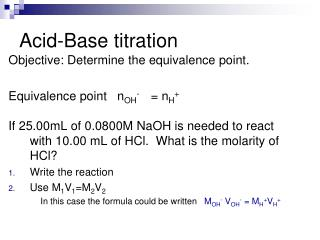DownloadDownload PresentationAcid-Base titration

# Acid-Base titration

Télécharger la présentation## Acid-Base titration

- - - - - - - - - - - - - - - - - - - - - - - - - - - E N D - - - - - - - - - - - - - - - - - - - - - - - - - - -
##### Presentation Transcript

1. Acid-Base titration Objective: Determine the equivalence point. Equivalence point nOH- = nH+ If 25.00mL of 0.0800M NaOH is needed to react with 10.00 mL of HCl. What is the molarity of HCl? • Write the reaction • Use M1V1=M2V2 In this case the formula could be written MOH- VOH- = MH+VH+

2. 4.4 Redox Reactions Tracking electrons in oxidation reduction reactions.

3. Oxidation-Reduction Reactions(Red-Ox) • A type of electrochemistry with reactions that involve a transfer of electronsbetween atoms. • The charge or oxidation number changes Ex:Fe2+ Fe 3+ + 1 e- In this red-ox ½ reaction the Iron (II) lost an electron to become Iron (III). • The electron does not just “go into space” so there must be a companion ½ reactionthat gains an electron to make the reaction balance.

4. Oxidation Number • The oxidation number of an element in a molecule is the charge that it would have if all shared electrons were assigned to the more electronegative elements in their bonds. • Draw the Lewis diagram for water • Assign the bonding electrons to the more En atoms. • Determine what the charge would be.

5. Rules for assigning oxidation numbers. • The oxidation state of an element is zero. • including all elemental forms of the elements (N2, P4, S8, O2, O3). • The oxidation state of a monatomic ion is the same as its charge. • Fe2+ oxidation number is 2+ • In compounds, Group 1 is +1, Group 2 is +2, Fluorine is -1 • Oxygen is usually assigned an oxidation state of -2 in its covalent compounds. • Exceptions to this rule include peroxides (compounds containing the group), where each oxygen is assigned an oxidation state of -1, as in hydrogen peroxide (H2O2). • Hydrogen is assigned an oxidation state of +1. • Metal hydrides are an exception In LiH, hydrogen has an oxidation state of -1. • The sum of the oxidation states must be zero for an electrically neutral compound. • For a polyatomic ion, the sum of the oxidation states must equal the charge of the ion.

6. Assigning Oxidation Numbers • Cl2 • Sodium Metal • Lead IV • NaF • CaCl2 • H2SO4 • CrO42- • NO3-

7. Red-ox reactions A spontaneous red-ox reaction can be used to perform electrical work. • Fuel Cells • Primary voltaic cells (alkaline batteries) • Storage Cells (car batteries)

8. Balancing Redox equations ClO3-(aq) + I-(aq) Cl-(aq) + I2 (s) The reaction occurs in an acidic solution. Split in to 2 half-reactions. An oxidation and a reduction.

9. Balancing ½ reactions • Balance the atoms of the element being oxidized or reduced. • Balance the oxidation number by balancing electrons. • Balance the charge. (add H+ if in acidic solution; add OH- if in basic solution) • Balance Hydrogen by adding H2O molecules. • Check to make sure that oxygen is balanced.

10. Balance the atoms of the element being oxidized or reduced. Balance the oxidation number by balancing electrons. Oxidation ½ add electrons to the right side Balance the charge. (add H+ if in acidic solution; add OH- if in basic solution) Balance Hydrogen by adding H2O molecules. Check to make sure that oxygen is balanced I-(aq)  I2(s)(in acidic solution)

11. Balance the atoms of the element being oxidized or reduced. Balance the oxidation number by balancing electrons. Reduction ½ adds e- to the left side. Balance the charge. (add H+ if in acidic solution; add OH- if in basic solution) Balance Hydrogen by adding H2O molecules. Check to make sure that oxygen is balanced ClO3-(aq)  Cl- (aq)(in acidic solution)

12. Combining ½ reactions • Combine so that the number of e- cancel. • Multiply by coefficients to accomplish this. • Add together and eliminate what appears on both sides.

13. The following reaction occurs in acidic solution:Fe2+ (aq) + MnO4- (aq)  Fe3+ (aq)+ Mn2+ (aq)

14. The following reaction occurs in basic solution:Cl2(g) + Cr(OH)3(s)  Cl- (aq)+ CrO42- (aq)